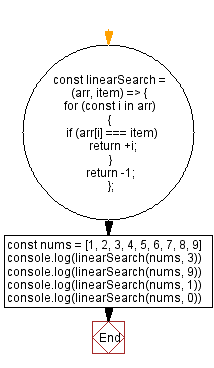# JavaScript - Linear search algorithm

## JavaScript Searching Algorithm: Exercise-2 with Solution

Write a JavaScript program to find the first index of a given element in an array using the linear search algorithm.

From Wikipedia -
In computer science, a linear search or sequential search is a method for finding an element within a list. It sequentially checks each element of the list until a match is found or the whole list has been searched.
A linear search runs in at worst linear time and makes at most n comparisons, where n is the length of the list. If each element is equally likely to be searched, then linear search has an average case of (n+1)/2 comparisons, but the average case can be affected if the search probabilities for each element vary. Linear search is rarely practical because other search algorithms and schemes, such as the binary search algorithm and hash tables, allow significantly faster searching for all but short lists.

Test Data:
([1, 2, 3, 4, 5, 6, 7, 8, 9], 3) -> 2
([1, 2, 3, 4, 5, 6, 7, 8, 9], 9) -> 8
([1, 2, 3, 4, 5, 6, 7, 8, 9], 1) -> 0
([1, 2, 3, 4, 5, 6, 7, 8, 9], 0) -> -1

Sample Solution:

JavaScript Code:

``````const linearSearch = (arr, item) => {
for (const i in arr)
{
if (arr[i] === item)
return +i;
}
return -1;
};
const nums = [1, 2, 3, 4, 5, 6, 7, 8, 9]
console.log(linearSearch(nums, 3))
console.log(linearSearch(nums, 9))
console.log(linearSearch(nums, 1))
console.log(linearSearch(nums, 0))
```
```

Sample Output:

```2
8
0
-1
```

Flowchart:Live Demo:

See the Pen javascript-searching-algorithm-exercise-2 by w3resource (@w3resource) on CodePen.

* To run the code mouse over on Result panel and click on 'RERUN' button.*

Improve this sample solution and post your code through Disqus

What is the difficulty level of this exercise?

Test your Programming skills with w3resource's quiz.

﻿